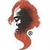0
Respuestas

Scatter series points with different color

Xavier Hahn hace 5 años en API actualizado por anonymous hace 5 años
I'm trying to make a graph with a few points, with some that should be a different color based on whether they are "outliers" or not. Basically, I display scatter points, and if a property on the list I'm binding the ScatterSeries to is "true", then the color of the point should be red, otherwise it should be black.

I've tried using the LinearColorAxis for this, but no luck yet. Here's the code (WPF) I have now:
<oxy:plot title="Repetitions">
<oxy:plot.axes>
<oxy:linearcoloraxis key="Outliers" position="Right" minimum="0" maximum="1" highcolor="Red" lowcolor="Black"></oxy:linearcoloraxis>
<oxy:linearaxis position="Bottom" title="Measurement"></oxy:linearaxis>
<oxy:plot.series>
<oxy:scatterseries itemssource="{Binding Item.Repetitions}" coloraxiskey="Outliers" datafieldx="RepetitionNbr" datafieldy="ExcessRayleighRatio" datafieldvalue="IsOutlierValue"></oxy:scatterseries>
</oxy:plot.series>
</oxy:plot.axes>
</oxy:plot>
The DataFieldValue does seem to be took (if I put a breakpoint in the property, I can see the binding working, the IsOutlierValue is a "double" property that returns 1.0 if I want the point "red", and 0.0 if I want it black. But the points don't change color.En revisión
Try changing Minimum=0.1 and Maximum=0.9 in your LinearColorAxis
The HighColor is used for values above the Maximum defined in the axis!And set Position = None if you don't need to see the color axis.+1
[Example("Colored outliers")]
public static PlotModel ColoredOutliers()
{
var plotModel1 = new PlotModel { Title = "Colored outliers" };
plotModel1.Axes.Add(new LinearColorAxis { Position = AxisPosition.None, Minimum = 0.1, Maximum = 0.9, HighColor = OxyColors.Red, LowColor = OxyColors.Black });
var series = new ScatterSeries();
series.Points.Add(new ScatterPoint(0, 0) { Value = 0 });
series.Points.Add(new ScatterPoint(1, 0) { Value = 0 });
series.Points.Add(new ScatterPoint(2, 1) { Value = 1 });
series.Points.Add(new ScatterPoint(3, -1) { Value = 1 });